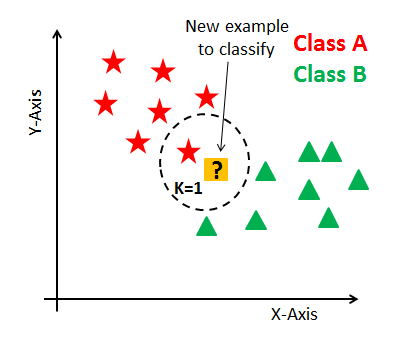# KNN Classification using Scikit-learnKNN Classification using Scikit-learn: Learn K-Nearest Neighbor(KNN) Classification and build a KNN classifier using Python Scikit-learn package.

Learn K-Nearest Neighbor(KNN) Classification and build a KNN classifier using Python Scikit-learn package.

K Nearest Neighbor(KNN) is a very simple, easy to understand, versatile, and one of the topmost machine learning algorithms. KNN used in a variety of applications such as finance, healthcare, political science, handwriting detection, image recognition, and video recognition. In Credit ratings, financial institutes will predict the credit rating of customers. In loan disbursement, banking institutes will predict whether the loan is safe or risky. In political science, classifying potential voters in two classes will vote or won’t vote. KNN algorithm used for both classification and regression problems. KNN algorithm based on the feature similarity approach.

In this tutorial, you are going to cover the following topics:

• K-Nearest Neighbor Algorithm
• How does the KNN algorithm work?
• Eager Vs Lazy learners
• How do you decide the number of neighbors in KNN?
• Curse of Dimensionality
• Classifier Building in Scikit-learn
• Pros and Cons
• How to improve KNN performance?
• Conclusion

## K-Nearest Neighbors

KNN is a non-parametric and lazy learning algorithm. Non-parametric means there is no assumption for underlying data distribution. In other words, the model structure determined from the dataset. This will be very helpful in practice where most of the real-world datasets do not follow mathematical theoretical assumptions. The lazy algorithm means it does not need any training data points for model generation. All training data used in the testing phase. This makes training faster and testing phase slower and costlier. The costly testing phase means time and memory. In the worst case, KNN needs more time to scan all data points, and scanning all data points will require more memory for storing training data.

## Data Science With Python Training | Python Data Science Course | Intellipaat

🔵 Intellipaat Data Science with Python course: https://intellipaat.com/python-for-data-science-training/In this Data Science With Python Training video, you...

## Applied Data Analysis in Python Machine Learning and Data Science | Scikit-Learn

Applied Data Analysis in Python Machine learning and Data science, we will investigate the use of scikit-learn for machine learning to discover things about whatever data may come across your desk.

## Data Science Projects | Data Science | Machine Learning | Python

Practice your skills in Data Science with Python, by learning and then trying all these hands-on, interactive projects, that I have posted for you.

## Data Science Projects | Data Science | Machine Learning | Python

Practice your skills in Data Science with Python, by learning and then trying all these hands-on, interactive projects, that I have posted for you.

## Data Science Projects | Data Science | Machine Learning | Python

Practice your skills in Data Science with Python, by learning and then trying all these hands-on, interactive projects, that I have posted for you.